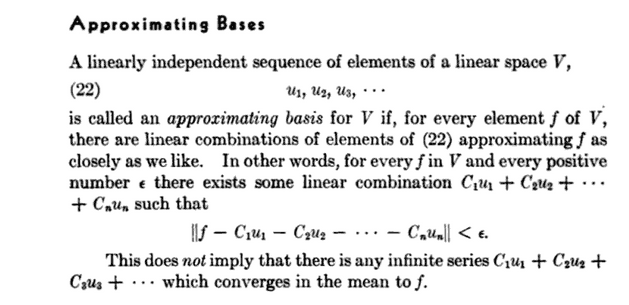# "Approximating Basis" -- Is there a contemporary term?

• I
• Ziva

#### Ziva

The term approximating basis is used by author Harry Floyd David is his book Fourier Series and Orthogonal Functions
on page 56:So I have looked in other books on functional analysis, harmonic analysis...and even on Google and I cannot find any other text reference that uses this term. This book is very sophisticated, but a bit dated and unfortunately has no bibliography. So my question is this:
What is the contemporary term for an approximating basis of a countably infinite dimensional linear space over ℝ(or ℂ) equipped with an inner product?

Last edited by a moderator:

•WWGD, Ziva and FactChecker
These are often called a Schauder basis.

https://en.m.wikipedia.org/wiki/Schauder_basis

Though note even Wikipedia doesn't totally believe that, as the section in Hilbert spaces on orthonormal bases just calls them a basis without further clarification

https://en.m.wikipedia.org/wiki/Hilbert_space
I am not sure that the text from the book attached to the OP indeed defines a Schauder basis. Note that the ##\epsilon##-close linear combination may depend on ##\epsilon##.

So, given ##f \in V## there need not exist a (unique) infinite sequence of scalars such that the associated series converges to ##f## in the norm of ##V##. (I think this is also what the remark in the book at the end refers to.) On the other hand, the definition of a Schauder basis does require this.

•Infrared
I am not sure that the text from the book attached to the OP indeed defines a Schauder basis. Note that the ##\epsilon##-close linear combination may depend on ##\epsilon##.

I agree. I think ##\{1,x,x^2,...\}## would be an approximating basis for ##C[0,1]## according to the OP's definition, but not a Schauder basis. Every continuous function on a compact interval can be uniformly approximated by polynomials (this is a theorem of Weierstrass), even without being analytic.

•S.G. Janssens
You're right, I missed that last part.

I guess in a Hilbert space the basis will be a Schauder basis if and only if it's at least mostly orthogonal. If the basis is orthogonal then it's clear adding future terms doesn't adjust your best choice of coefficients for the earlier terms.

If e.g. none of the terms are orthogonal, then if you think about it in terms of an orthogonalized version of the basis, every time you add a new term you're basically adjusting all the older coefficients in the orthogonal basis, so you can't possibly stabilize on the right set. If the vectors are close enough to orthogonal then this is maybe not a problem.

As an extreme example, if you can partition it into a disjoint union of finite subsets such that every vector is orthogonal to everyone outside of its partition, then it will still be a Schauder basis I think. Or if you pick an orthogonal basis, and then add ##a_n v_1## to each ##v_n## for some choice of ##a_n## that converges sufficiently quickly to 0,I think you can just cleverly pick the coefficient on the first vector to cancel out all the future adjustments the latter vectors will make.

Here is a bit more about the definition in the copied book text in the OP.
1. Assume that ##V## is an infinite-dimensional real or complex normed linear space. Then ##V## has an approximating basis if and only if ##V## is separable. (##\Longrightarrow## is not difficult, and ##\Longleftarrow## is a bit more difficult because, starting from a countable dense subset, you need to extract a linearly independent subset.)

2. Assume that ##V## is also complete, so it is a Banach space. If ##V## has a Schauder basis, then ##V## is separable. The converse is false, but this is difficult. Enflo gave the first counterexample in Acta Mathematica 130, 1973, p. 309 - 317.

3. Finally, assume that ##V## is also a Hilbert space. Then separability is equivalent to existence of an orthonormal basis, which is clearly a Schauder basis.
So, in the case of a Hilbert space, existence of an approximating basis IS equivalent to existence of a Schauder basis. In the case of a Banach space that is not an inner product space, the situation is more difficult and as outlined above.

Last edited:
•Keith_McClary
These are often called a Schauder basis.
The last line in the text cited in #1 shows that it is not a Schauder basis

Then separability is equivalent to existence of an orthonormal basis
In Hilbert space orthonormal basis exists even in the nonseparable case see for example Yosida Functional Analysis. Orthonormal basis in Hilbert space is not exactly the same as Schauder basis. Just for separable case any orthonormal basis is a Schauder basis

In Hilbert space orthonormal basis exists even in the nonseparable case see for example Yosida Functional Analysis. Orthonormal basis in Hilbert space is not exactly the same as Schauder basis. Just for separable case any orthonormal basis is a Schauder basis
With post #1 in mind, in post #7 I consider orthonormal basis to be countable. This is the more elementary definition. For the OP: See for example at the top of p. 28 of Basic Classes of Linear Operators by Gohberg, Goldberg, and Kaashoek, Birkhäuser, 2003. Of course wrobel is right that extensions and generalizations are possible.

Then, in a Hilbert space, there is the equivalence between: separability and existence of an approximating / orthonormal / Schauder basis. For the OP: See Theorem 17.1 on p. 35 of the above book.

As a side remark, I find this book a very nice introductory read, in line and probably at the level of the book mentioned in post #1.